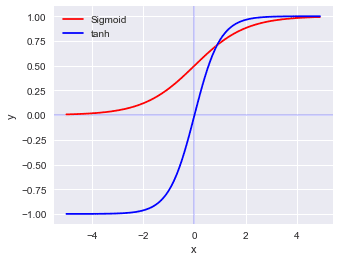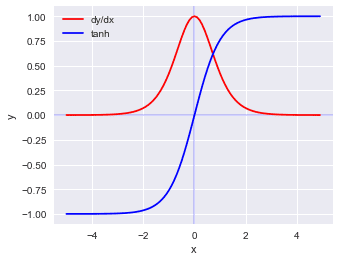search
Search
Map of Data Science
Guest 0reps
exit_to_appLog out
Map of data science
Thanks for the thanks!
close
Outline
chevron_left Activation functions
Cancel
Post
account_circle
Profile
exit_to_app
Sign out
search
keyboard_voice
close
Searching Tips
Search for a recipe:
"Creating a table in MySQL"
Search for an API documentation: "@append"
Search for code: "!dataframe"
Apply a tag filter: "#python"
Useful Shortcuts
/ to open search panel
Esc to close search panel
to navigate between search results
d to clear all current filters
Enter to expand content previewDoc SearchCode Search BetaSORRY NOTHING FOUND!
mic
Start speaking...Voice search is only supported in Safari and Chrome.
Shrink
Navigate to
A
A
brightness_medium
share
arrow_backShareTwitterFacebook
chevron_left Activation functions
check_circle
Mark as learned
thumb_up
0
thumb_down
0
chat_bubble_outline
0
auto_stories new
settings

# Comprehensive Guide on tanh

Machine Learning
chevron_right
Neural Networks
chevron_right
Activation functions
schedule Jul 1, 2022
Last updated
local_offer
Tags
expand_more
map
Check out the interactive map of data science

The $\mathrm{tanh}$ curve looks similar to the sigmoid curve in that the shape is s-like. $\mathrm{tanh}$ takes in any real value as input, and outputs a value from $-1$ to $1$.

The following is a graph of $\mathrm{tanh}$ and sigmoid function:Mathematically, we can represent $\mathrm{tanh}(x)$ like so:

$$\mathrm{tanh}(x)=\frac{e^x-e^{-x}}{e^x+e^{-x}}$$

# Derivative

What makes $\mathrm{tanh}$ appealing as an activation function is that the derivative of $\mathrm{tanh}$ is clean:

$$\frac{d}{dx}\mathrm{tanh}(x)=1-\mathrm{tanh}(x)^2$$

The graph of the derivative of $\mathrm{tanh}(x)$ looks like the following:# Code

The code used to generate the plot is as follows:

 import numpy as npimport matplotlib.pyplot as pltplt.style.use('seaborn-whitegrid')plt.style.use('seaborn')def sigmoid(x): return 1 / (1 + np.exp(-x))x_tanh = np.arange(-5, 5, 0.1)y_tanh = np.tanh(x)plt.figure(figsize=(5,4))plt.xlabel("x")plt.ylabel("y")plt.axhline(0, color="blue", alpha=0.2)plt.axvline(0, color="blue", alpha=0.2)x_sigmoid = np.arange(-5, 5, 0.1)y_sigmoid = sigmoid(x)plt.plot(x_sigmoid, y_sigmoid, color="red", label="Sigmoid")plt.plot(x_tanh, y_tanh, color="blue", label="tanh")plt.legend()